# JP5339784B2 - Zoom lens and imaging apparatus having the same - Google Patents

## Info

Publication number
JP5339784B2
JP5339784B2 JP2008145623A JP2008145623A JP5339784B2 JP 5339784 B2 JP5339784 B2 JP 5339784B2 JP 2008145623 A JP2008145623 A JP 2008145623A JP 2008145623 A JP2008145623 A JP 2008145623A JP 5339784 B2 JP5339784 B2 JP 5339784B2
Authority
JP
Japan
Prior art keywords
lens
lt
zoom lens
zoom
lens unit
Prior art date
Legal status (The legal status is an assumption and is not a legal conclusion. Google has not performed a legal analysis and makes no representation as to the accuracy of the status listed.)
Expired - Fee Related
Application number
JP2008145623A
Other languages
Japanese (ja)
Other versions
JP2009294304A5 (en
JP2009294304A (en
Inventor

Original Assignee
キヤノン株式会社
Priority date (The priority date is an assumption and is not a legal conclusion. Google has not performed a legal analysis and makes no representation as to the accuracy of the date listed.)
Filing date
Publication date
Application filed by キヤノン株式会社 filed Critical キヤノン株式会社
Priority to JP2008145623A priority Critical patent/JP5339784B2/en
Publication of JP2009294304A publication Critical patent/JP2009294304A/en
Publication of JP2009294304A5 publication Critical patent/JP2009294304A5/ja
Application granted granted Critical
Publication of JP5339784B2 publication Critical patent/JP5339784B2/en
Application status is Expired - Fee Related legal-status Critical
Anticipated expiration legal-status Critical

• 239000000463 materials Substances 0 abstract 3
• 230000003287 optical Effects 0 abstract 3
• 238000009740 moulding (composite fabrication) Methods 0 abstract 2

## Images

•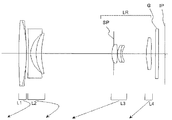•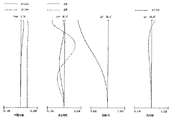••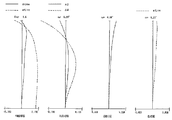•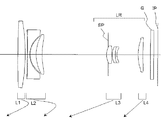•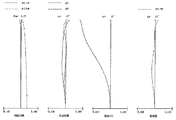•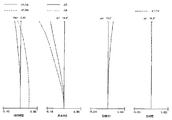•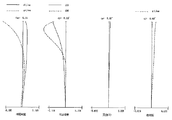•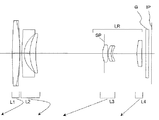•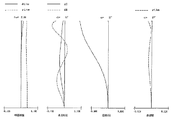••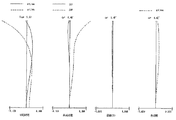•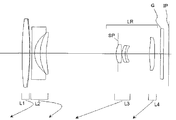•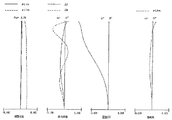•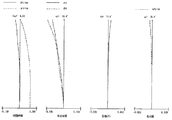•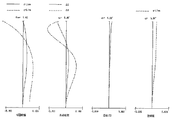•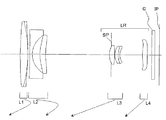•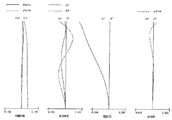•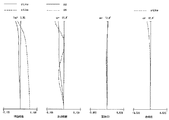•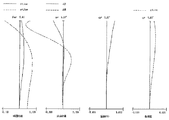•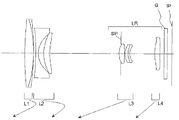•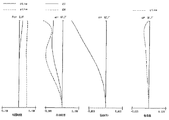•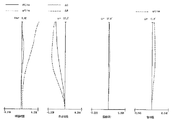•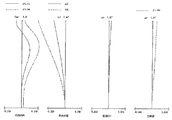•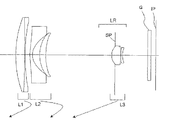•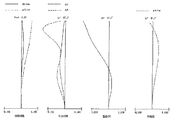•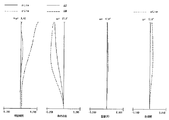•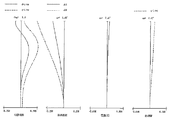•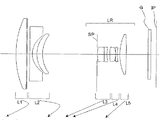•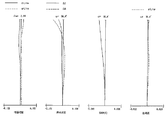•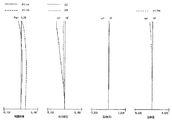•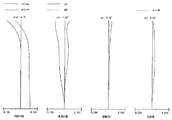•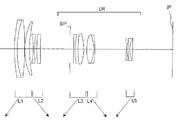•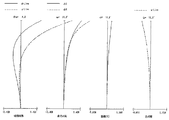•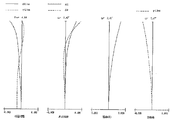•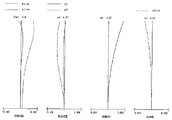•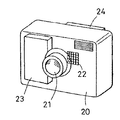## Classifications

• GPHYSICS
• G02OPTICS
• G02BOPTICAL ELEMENTS, SYSTEMS, OR APPARATUS
• G02B15/00Optical objectives with means for varying the magnification
• G02B15/14Optical objectives with means for varying the magnification by axial movement of one or more lenses or groups of lenses relative to the image plane for continuously varying the equivalent focal length of the objective
• G02B15/16Optical objectives with means for varying the magnification by axial movement of one or more lenses or groups of lenses relative to the image plane for continuously varying the equivalent focal length of the objective with interdependent non-linearly related movements between one lens or lens group, and another lens or lens group
• G02B15/163Optical objectives with means for varying the magnification by axial movement of one or more lenses or groups of lenses relative to the image plane for continuously varying the equivalent focal length of the objective with interdependent non-linearly related movements between one lens or lens group, and another lens or lens group having a first movable lens or lens group and a second movable lens or lens group, both in front of a fixed lens or lens group
• G02B15/167Optical objectives with means for varying the magnification by axial movement of one or more lenses or groups of lenses relative to the image plane for continuously varying the equivalent focal length of the objective with interdependent non-linearly related movements between one lens or lens group, and another lens or lens group having a first movable lens or lens group and a second movable lens or lens group, both in front of a fixed lens or lens group having an additional fixed front lens or group of lenses
• G02B15/173Optical objectives with means for varying the magnification by axial movement of one or more lenses or groups of lenses relative to the image plane for continuously varying the equivalent focal length of the objective with interdependent non-linearly related movements between one lens or lens group, and another lens or lens group having a first movable lens or lens group and a second movable lens or lens group, both in front of a fixed lens or lens group having an additional fixed front lens or group of lenses arranged +-+
• GPHYSICS
• G02OPTICS
• G02BOPTICAL ELEMENTS, SYSTEMS, OR APPARATUS
• G02B15/00Optical objectives with means for varying the magnification
• G02B15/14Optical objectives with means for varying the magnification by axial movement of one or more lenses or groups of lenses relative to the image plane for continuously varying the equivalent focal length of the objective
• G02B15/142Optical objectives with means for varying the magnification by axial movement of one or more lenses or groups of lenses relative to the image plane for continuously varying the equivalent focal length of the objective having two groups only

## Abstract

A zoom lens system includes, in order from an object side to an image side, a first lens unit having positive optical power, a second lens unit having negative optical power, and a rear unit including at least one lens unit. During zooming, at least two lens units move so that intervals between the lens units adjacent to each other change. In such a zoom lens system, the second lens unit consists of a negative lens component formed of at least one material and a positive lens element, which are arranged in the stated order from the object side to the image side. A refractive index and an Abbe number of the at least one material forming the negative lens component and a refractive index of a material forming the positive lens element are appropriately set, whereby high optical performance is obtained.

## Description

The present invention relates to a zoom lens and an image pickup apparatus having the same, and is suitable for an image pickup apparatus using a solid-state image pickup device such as a video camera, an electronic still camera, a broadcast camera, a surveillance camera, or a silver salt photograph camera. It is a thing.

In recent years, imaging devices such as a video camera using a solid-state imaging device, a digital still camera, a broadcasting camera, a surveillance camera, and a camera using a silver salt film have been improved in function, and the entire device has been downsized.

A photographing optical system used therefor is required to be a zoom lens having a short overall lens length, a compact size, a high zoom ratio (high zoom ratio), and a high resolution.

In addition, a retractable zoom lens is desired that includes a configuration in which the distance between the lens groups is reduced to a different distance from the shooting state during non-shooting to reduce the overall thickness (length in the optical axis direction) of the image pickup apparatus. Has been.

As a zoom lens that meets these requirements, zoom is made up of first, second, and third lens groups having positive, negative, and positive refractive powers in order from the object side to the image side, and zooming is performed by moving each lens group. A lens is known (Patent Document 1).

In addition, a positive lead type zoom lens having a rear group including a lens unit having a positive refractive power, a lens unit having a negative refractive power, and a subsequent one or more lens units in order from the object side to the image side is known. (Patent Documents 2 and 3).

Patent Documents 2 and 3 disclose a zoom lens that is composed of four lens groups of positive, negative, positive, and positive refractive power lenses in order from the object side to the image side, and performs zooming by moving each lens group. ing.

In particular, Patent Document 3 discloses a zoom lens in which the second lens group is composed of two lenses, a negative lens and a positive lens, and the refractive power of the constituent elements is appropriately set to reduce the size of the entire system. ing.

In recent years, translucent ceramics have been developed, and photographing optical systems using them as optical materials are known. Translucent ceramics have a higher refractive index than optical glass, and are excellent in hardness and strength. An imaging apparatus that uses this property to reduce the thickness of the entire lens system is known (Patent Document 4).

In Patent Document 4, a lens thickness is made thin by using translucent ceramics as a material of a negative lens of a cemented lens in which a positive lens and a negative lens are bonded together, thereby reducing the size of the entire lens system.
JP 2005-106925 A JP 2005-338740 A JP 2007-171371 A JP 2006-84887 A

In recent years, there is a strong demand for a zoom lens used in an imaging apparatus that has a high zoom ratio and that the entire lens system is small.

In general, in order to reduce the size of a zoom lens, the number of lenses may be reduced while increasing the refractive power of each lens group constituting the zoom lens.

However, in such a zoom lens, the lens thickness increases, the effect of shortening the lens system becomes insufficient, and correction of various aberrations becomes difficult.

For this reason, in order to achieve a high zoom ratio and downsizing of the entire lens system, it is important to appropriately set the zoom type, the refractive power of each lens group, the lens configuration constituting each lens group, and the like.

In general, optical glass has a characteristic that when the refractive index increases, the Abbe number decreases and the dispersion increases.

On the other hand, ceramics are known to have a higher refractive index than optical glass having the same Abbe number.

Use of ceramics having such properties as an optical material is advantageous for aberration correction and downsizing of the entire optical system.

However, it is difficult to obtain high optical performance over the entire zoom range while reducing the size of the entire system even if a lens made of ceramics is simply used for the zoom lens.

In particular, in the positive lead type zoom lens described above, in order to achieve a high zoom ratio while reducing the effective diameter of the first lens unit and reducing the size of the entire system, the lens configuration in the second lens unit is appropriately set. It becomes important to do. For example, it is important to select an appropriate material for the lenses constituting the second lens group and to configure the second lens group with as few lenses as possible.

In a positive lead type zoom lens, if the lens configuration of the second lens group is inappropriate, it is very difficult to obtain high optical performance over the entire zoom range while reducing the size of the entire system.

SUMMARY OF THE INVENTION An object of the present invention is to provide a small zoom lens capable of obtaining a high zoom ratio and high optical performance over the entire zoom range, and an imaging apparatus having the same.

The zoom lens according to the present invention includes, in order from the object side to the image side, a first lens group having a positive refractive power, a second lens group having a negative refractive power, and a third lens group having a positive refractive power. During zooming from the telephoto end to the telephoto end, the first lens group moves so as to be positioned closer to the object side at the telephoto end than at the wide-angle end, and the distance between the first lens group and the second lens group increases. A zoom lens in which a distance between the second lens group and the third lens group is reduced , and the second lens group includes a negative lens and a positive lens in order from the object side to the image side. When the refractive index and Abbe number for the line are N2n and ν2n, respectively, and the refractive index for the d-line of the positive lens is N2p,
2.45 <N2n- (9.3 × 10 −5 × ν2n 2 −1.70 × 10 −2 × ν2n) <3.00
5 <ν2n <80
1.98 <(N2n + N2p) / 2 <2.25
It is characterized by satisfying the following conditions.

According to the present invention, it is possible to obtain a small zoom lens having a high zoom ratio and high optical performance over the entire zoom range, and an imaging apparatus having the same.

Embodiments of the zoom lens of the present invention and an image pickup apparatus having the same will be described below.

The zoom lens according to the present invention includes, in order from the object side to the image side, a first lens group having a positive refractive power, a second lens group having a negative refractive power, and a rear group including one or more lens groups. .

At the time of zooming, at least the second lens group is moved.

For example, the rear lens group includes a third lens unit having a positive refractive power. Alternatively, the lens unit includes a third lens group having a positive refractive power and a fourth lens group having a positive refractive power in order from the object side to the image side.

Alternatively, the lens unit includes a third lens group having a positive refractive power, a fourth lens group having a positive refractive power, and a fifth lens group having a positive refractive power in order from the object side to the image side.

In the present invention, the rear group may be composed of four or more lens groups.

FIG. 1 is a cross-sectional view (lens cross-sectional view) of a main part at the wide angle end (short focal length end) of the zoom lens according to the first exemplary embodiment. Figures 2-4, respectively, at the wide angle end of the zoom lens in Example 1, is a diagram of aberrations in the intermediate focal length, a telephoto end (long focal length end).

FIG. 5 is a cross-sectional view of the main part of the zoom lens of Embodiment 2 at the wide-angle end. 6 to 8 are aberration diagrams of the zoom lens according to Example 2 at the wide-angle end, the intermediate focal length, and the telephoto end, respectively.

FIG. 9 is a lens cross-sectional view of the lens at the wide-angle end of the zoom lens according to the third exemplary embodiment. 10 to 12 are aberration diagrams of the zoom lens of Example 3 at the wide-angle end, the intermediate focal length, and the telephoto end, respectively.

FIG. 13 is a cross-sectional view of the main part of the zoom lens of Embodiment 4 at the wide-angle end. 14 to 16 are aberration diagrams of the zoom lens of Example 4 at the wide-angle end, the intermediate focal length, and the telephoto end, respectively.

FIG. 17 is a lens cross-sectional view of the zoom lens of Example 5 at the wide-angle end. 18 to 20 are aberration diagrams of the zoom lens of Example 5 at the wide-angle end, the intermediate focal length, and the telephoto end, respectively.

FIG. 21 is a lens cross-sectional view of the zoom lens of Example 6 at the wide-angle end. 22 to 24 are aberration diagrams of the zoom lens of Example 6 at the wide-angle end, the intermediate focal length, and the telephoto end, respectively.

FIG. 25 is a lens cross-sectional view of the zoom lens of Example 7 at the wide-angle end. 26 to 28 are aberration diagrams at the wide-angle end, the intermediate focal length, and the telephoto end, respectively, of the zoom lens according to the seventh embodiment.

FIG. 29 is a lens cross-sectional view of the zoom lens of Example 8 at a wide angle end. 30 to 32 are aberration diagrams at the wide-angle end, the intermediate focal length, and the telephoto end, respectively, of the zoom lens according to the eighth embodiment.

FIG. 33 is a lens cross-sectional view of the zoom lens of Example 9 at the wide-angle end. 34 to 36 are aberration diagrams at the wide-angle end, the intermediate focal length, and the telephoto end, respectively, of the zoom lens according to the ninth embodiment.

FIG. 37 is a schematic diagram of a main part of a camera (image pickup apparatus) including the zoom lens of the present invention.

The zoom lens of each embodiment is an imaging lens system used in an imaging apparatus such as a video camera or a digital camera. In the lens cross-sectional view, the left side is the object side (front), and the right side is the image side (rear).

When the zoom lens of each embodiment is used as a projection lens such as a projector, the left side is the screen and the right side is the projected image.

In the lens cross-sectional view, i indicates the order of the lens groups from the object side, and Li is the i-th lens group. LR is a rear group including one or more lens groups.

In the lens cross-sectional view, L1 is a first lens group having a positive refractive power, L2 is a second lens group having a negative refractive power, L3 is a third lens group having a positive refractive power, and L4 is a fourth lens having a positive refractive power. The group L5 is a fifth lens group having a positive refractive power.

SP is an aperture stop (F-number determining stop). The aperture stop SP is disposed between the object-side vertex of the lens disposed closest to the object side of the third lens unit L3 in Embodiments 1 to 7, and the intersection of the object-side surface and the outer periphery of the lens.

In Examples 8 and 9, the third lens unit L3 is disposed on the object side.

G is an optical block corresponding to an optical filter, a face plate, a quartz low-pass filter, an infrared cut filter, or the like.

IP is an image plane. When used as an imaging optical system of a video camera or a digital still camera, an imaging plane of a solid-state imaging device (photoelectric conversion device) such as a CCD sensor or a CMOS sensor is placed.

Further, when used as an imaging optical system of a silver salt film camera, it corresponds to a film surface.

In the aberration diagrams, d and g are d-line and g-line, respectively, ΔM and ΔS are meridional image surface, sagittal image surface, and lateral chromatic aberration are represented by g-line. ω is a half angle of view, and Fno is an F number.

In the following embodiments, the wide-angle end and the telephoto end refer to zoom positions when the zoom lens unit is positioned at both ends of the range in which the mechanism can move on the optical axis.

The arrows indicate the movement trajectory of each lens unit during zooming from the wide-angle end to the telephoto end.

During zooming from the wide-angle end to the telephoto end, the first lens unit L1 moves so as to be positioned closer to the object side at the telephoto end than at the wide-angle end.

The second lens group moves so that the distance between the first lens group L1 and the second lens group L2 is increased.

The third lens unit L3 moves so that the distance between the second lens unit L2 and the third lens unit L3 is small.

The zoom lens according to each embodiment includes, in order from the object side to the image side, a first lens unit L1 having a positive refractive power, a second lens unit L2 having a negative refractive power, and a rear unit LR including one or more lens units. ing. By using a material with a high refractive index made of translucent ceramics while constituting the second lens unit L2 with two lenses, a small zoom lens having a high zoom ratio and high optical performance is achieved. doing.

The second lens unit L2 includes a biconcave lens or a negative meniscus lens having a convex object side and a positive meniscus lens having a convex object side. By using a material with a high refractive index for this negative lens, the occurrence of various aberrations such as coma and curvature of field is reduced by loosening the curvature of each lens surface, and the optical element itself is made thinner. I am trying.

At least one surface of the negative lens is aspherical. In this case, when the object side surface is an aspherical shape, the aspherical shape is a shape that increases the negative refractive power toward the off-axis, thereby correcting the curvature of field that goes under the off-axis.

Further, when the image side surface is aspherical, in order to cancel barrel distortion caused by the object side surface, the negative power decreases toward the off-axis.

The aspherical surface may be formed directly on the base material (lens) or may be formed by laminating a resin material (replica) on the base material.

On the other hand, the meniscus positive lens in the second lens unit L2 requires a material having higher dispersion than the negative lens in order to correct chromatic aberration in the second lens unit.

As described above, optical glass tends to be highly dispersed as the refractive index increases. Ceramics and oxide single crystals and polycrystals have relatively low dispersion even when the refractive index increases. Therefore, by using these materials, it is possible to reduce the size of the entire system while correcting chromatic aberration.

In general, optical glass is a graph in which the refractive index is taken to be a large value on the vertical axis and the Abbe number is taken to be a large value on the left side on the horizontal axis (hereinafter referred to as “nd-νd diagram”). When mapped up, it is known to be distributed along almost several straight lines. In general, when the refractive index of the optical glass increases, the Abbe number decreases and the dispersion increases.

On the other hand, among single crystals and polycrystals of ceramics and oxides having high light transmittance in the visible light region, the relationship between the refractive index and the Abbe number is different from that of ordinary optical glass in the nd-νd diagram described above. Are known to exist in different regions. That is, a material having a higher refractive index than that of optical glass having the same Abbe number is known.

In the present invention, such a material is used for at least one material of the positive lens and the negative lens of the second lens unit L2.

In each embodiment, the refractive index and Abbe number of at least one material of the negative lens constituting the second lens unit L2 with respect to the d-line are N2n and ν2n, respectively. The refractive index with respect to the d-line of the positive lens constituting the second lens unit L2 is N2p. At this time,
2.45 <N2n− (9.3 × 10 −5 × ν2n 2 −1.70 × 10 −2 × ν2n) <3.00 (1)
5 <ν2n <80 (2)
1.98 <(N2n + N2p) / 2 <2.25 (3)
The conditions are satisfied.

Next, the technical meaning of each conditional expression described above will be described.

Conditional expression (1) defines the relationship between the refractive index and the Abbe number at the d-line of the material of the negative lens of the second lens L2. If the refractive index falls below the lower limit of conditional expression (1) and the refractive index of the negative lens decreases, the refractive power also decreases and the amount of movement of the second lens unit L2 increases to obtain a sufficient zoom ratio, and the lens system becomes larger. Come.

Further, if the refractive index of the negative lens material increases beyond the upper limit of conditional expression (1), the effect of correcting the Petzval sum in the negative direction becomes weak and the image surface falls to the under side, which is not good.

Conditional expression (2) defines the Abbe number in the d-line of the material of the negative lens of the second lens unit L2. If the Abbe number is below the lower limit of the conditional expression (2), it is not possible to select a higher dispersion material as the positive lens material in order to correct the chromatic aberration in the second lens unit L2, and in particular, the magnification at the wide angle end. Correction of chromatic aberration becomes difficult. Also, if the negative lens becomes low dispersion exceeding the upper limit of conditional expression (2), the lateral chromatic aberration at the wide-angle end will be overcorrected, which is not good.

Conditional expression (3) defines the average refractive index of the material of the lenses constituting the second lens unit L2. When the average refractive index is below the lower limit of the conditional expression (3), the curvature of the lens surfaces of the negative lens and the positive lens becomes small, large high-order aberrations occur on each lens surface, and the optical performance deteriorates.

Further, the thickness (thickness) of each lens becomes longer, and the length of the entire second lens unit L2 on the optical axis increases. Further, if the average refractive index increases beyond the upper limit of the conditional expression (3), the effect of correcting the Petzval sum in the minus direction becomes weak, and the image plane characteristic falls to the under side, which is not good.

More preferably, the numerical ranges of conditional expressions (2) and (3) should be set as follows.

30 <ν2n <55 (2a)
2.00 <(N2n + N2p) / 2 <2.20 (3a)
As described above, by configuring each element, in each embodiment, the second lens group can be configured by two lenses while having a high zoom ratio and high optical performance. Miniaturization has been achieved.

By satisfying the above conditions, a zoom lens having a high zoom ratio and high optical performance over the entire zoom range is obtained.

In each embodiment, in order to further reduce aberrations during zooming while reducing aberration variation during zooming, one or more of the following conditions must be satisfied. good.

The distance from the most object side surface of the second lens unit L2 to the most image side surface is defined as D2. The focal lengths at the wide-angle end and the telephoto end of the zoom lens are fw and ft, respectively.

The Abbe number at the d-line of the positive lens of the second lens unit L2 is ν2p.

The focal lengths of the negative lens and the positive lens of the second lens unit L2 are f2n and f2p, respectively.

The lateral magnifications at the wide-angle end and the telephoto end of the second lens unit L2 are β2w and β2t, respectively.

The rear group LR includes a third lens unit L3 having a positive refractive power, and the lateral magnifications at the wide-angle end and the telephoto end of the third lens unit L3 are β3w and β3t, respectively.

The focal lengths of the first lens unit L1 and the second lens unit L2 are f1 and f2, respectively.

At this time, 0.05 <D2 / fw <1.2 (4)
1.98 <ν2n / ν2p <3.2 (5)
−0.4 <f2n / f2p <−0.2 (6)
2.8 <ft / fw <10 (7)
1.5 <| β2t / β2w | <3.2 (8)
0.3 <| β3t / β3w | <7.1 (9)
−2.5 <f2 / fw <−0.5 (10)
−6.8 <f1 / f2 <−1.7 (11)
It is preferable to satisfy one or more of the following conditions.

Next, the technical meaning of each conditional expression will be described.

Conditional expression (4) defines the thickness of the second lens unit L2 on the optical axis. If the thickness of the second lens unit L2 is less than the lower limit of the conditional expression (4), the negative lens and the positive lens interfere with each other, making it difficult to give a curvature of a predetermined refractive power to the lens surface. It becomes difficult to perform well.

In particular, lateral chromatic aberration and field curvature at the wide-angle end, and coma at the telephoto end are insufficiently corrected. On the other hand, if the thickness of the second lens unit L2 exceeds the upper limit of the conditional expression (4), the second lens unit L2 becomes too thick and it becomes difficult to shorten the retracted length when the retracting method is used.

Conditional expression (5) defines the relationship between the Abbe numbers in the d-line of the materials of the negative lens and the positive lens of the second lens unit L2. If the ratio of the Abbe number in the d-line of the negative lens and the positive lens material of the second lens unit L2 is below the lower limit of the conditional expression (5), the lateral chromatic aberration at the wide-angle end becomes insufficiently corrected. The thickness of the two lens unit L2 increases. Further, if the ratio of the Abbe number in the d-line of the material of the negative lens and the positive lens in the second lens unit L2 exceeds the upper limit of the conditional expression (5), the lateral chromatic aberration at the wide angle end becomes overcorrected.

Conditional expression (6) defines the ratio of the refractive power (reciprocal of focal length) of the negative lens and the positive lens of the second lens unit L2. When the absolute value of the focal length of the negative lens becomes longer than the focal length of the positive lens and falls below the lower limit of the conditional expression (6), the front lens diameter (first lens unit L1) increases and the entire system can be downsized. It becomes difficult. Further, when the absolute value of the focal length of the negative lens becomes shorter than the focal length of the positive lens and exceeds the upper limit of the conditional expression (6), various aberrations that occur off-axis at the wide-angle end become large, especially at the wide-angle end. Correction of lateral chromatic aberration and distortion is difficult.

Conditional expression (7) relates to the zoom ratio of the entire system. Conditional expression (7) clarifies the zoom range of each embodiment, has a high zoom ratio when using a predetermined material, has high optical performance, and achieves the most effective miniaturization of the entire system. The range that can be used is specified. Here, the zoom ratio depends on the refracting power of each lens group arranged during alteration, the movement range during zooming, and the like.

Conditional expression (8) and conditional expression (9) define an appropriate range of zooming action of the second lens unit L2 and the third lens unit L3. If the lower limit of conditional expression (8) is exceeded or the upper limit of conditional expression (9) is exceeded, the zooming action of the third lens unit L3 becomes too strong to correct various aberrations when trying to obtain a high zoom ratio. It becomes difficult, and the sensitivity at the time of manufacturing becomes high, so it is not good.

On the other hand, if the upper limit of conditional expression (8) is exceeded or the lower limit of conditional expression (9) is exceeded, the zooming action of the second lens unit L2 becomes too strong when trying to obtain a high zoom ratio, and various aberrations occur. It is not good because correction becomes difficult and the sensitivity in manufacturing increases. Further, by giving a large zooming share to the third lens unit L3, the second lens unit L2 moves with a convex locus on the image side during zooming.

Compared with the case where the second lens unit L2 moves linearly to the image side, it is less necessary to secure a space for moving the second lens unit L2 to the image side. The position can be arranged closer to the aperture stop SP. As a result, it becomes easy to keep the front lens diameter small.

Conditional expression (10) defines the ratio of the focal length of the second lens unit L2 to the focal length of the entire system at the wide angle end. If the focal length of the second lens unit L2 falls below the lower limit of the conditional expression (10) and the refractive power becomes weak, the movement amount of the second lens unit L2 increases to obtain a sufficient zoom ratio, and the entire system increases in size. come. Further, when the focal length of the second lens unit L2 exceeds the upper limit of the conditional expression (10) and the refractive power becomes strong, it becomes difficult to correct coma at the telephoto end. Furthermore, the thickness deviation ratio of the negative lens in the second lens unit L2 becomes large, and manufacturing becomes difficult. Further, since the thickness of each lens becomes longer due to the tight curvature of the lens surface, the entire thickness of the second lens unit L2 increases.

Conditional expression (11) defines the ratio of the focal lengths of the first lens unit L1 and the second lens unit L2. If the lower limit of conditional expression (11) is not reached, the F number at the telephoto end will increase, which is not good. On the other hand, if the upper limit of conditional expression (11) is exceeded, the front lens diameter will increase and the entire system will become larger, which is not good.

More preferably, the numerical ranges of the conditional expressions (4) to (11) are set as follows.

0.78 <D2 / fw <1.10 (4a)
2.0 <ν2n / ν2p <3.15 (5a)
−0.39 <f2n / f2p <−0.22 (6a)
5.8 <ft / fw <9 (7a)
1.6 <| β2t / β2w | <2.1 (8a)
4.3 <| β3t / β3w | <7.1 (9a)
-2.2 <f2 / fw <-1.6 (10a)
−6.1 <f1 / f2 <−4.5 (11a)
Next, the lens configuration of each embodiment of the zoom lens of the present invention will be described.

In Example 1 of FIG. 1, in order from the object side to the image side, a first lens unit L1 having a positive refractive power, a second lens unit L2 having a negative refractive power, a third lens unit L3 having a positive refractive power, The fourth lens unit L4 having a refractive power of 5 mm.

During zooming from the wide-angle end to the telephoto end, the first lens unit L1 moves along a locus convex to the image side, and the second lens unit L2 moves along a locus convex to the image side. Further, during zooming from the wide-angle end to the telephoto end, the third lens unit L3 moves to the object side to perform main zooming, and the fourth lens unit L4 moves to the object side to change the image plane due to zooming. Reduced (corrected). By moving the first lens unit L1 from the wide-angle end to a substantially intermediate position in the zoom range toward the image side, the distance from the aperture stop SP is shortened to reduce the diameter of the front lens.

The first lens group includes, in order from the object side, a positive lens (first optical element) (a refracting surface on the image side has a stronger positive power than a refracting surface on the object side) and a negative lens joined to the positive lens. A composite optical element having an optical element having a refractive power (second optical element) is provided. The second optical element is preferably made of a resin material. As described above, the point that the first lens group is composed of a positive lens and a composite optical element having an optical element having negative refractive power joined thereto is described in Examples 3, 4, 5, and 6 described later. , 8 is the same.

In this embodiment, the composite optical element is composed of a first optical element and a second optical element G12 (a plurality of optical elements may be joined) joined to the first optical element G11. The first optical element G11 and the second optical element (preferably an auxiliary lens made of resin) G12 bonded to the first optical element satisfy the following relationship.

(A) The thickness of the first optical element on the optical axis is at least three times (preferably at least five times) the thickness of the second optical element on the optical axis. Here, the thickness of the first optical element on the optical axis is preferably 100 times or less the thickness of the second optical element on the optical axis.

(A) The absolute value of the refractive power (the reciprocal of the focal length) of the first optical element is at least twice (preferably at least 2.5 times) the absolute value of the refractive power of the second optical element. Here, the absolute value of the refractive power (the reciprocal of the focal length) of the first optical element is 50 times or less (preferably 40 times or less) the absolute value of the refractive power of the second optical element.

The second lens unit L2 includes, in order from the object side, a biconcave negative lens and a meniscus positive lens convex on the object side. A material such as ceramics having a refractive index N2n of 1.97500 and an Abbe number of ν2n of 39.5 is used for the negative lens. Since the negative lens material has a high refractive index, if the lens surface has the same curvature, the occurrence of aberration can be suppressed even if the refractive power of the negative lens is increased. For this reason, it is easy to increase the zoom ratio, and if the magnification is the same, the curvature of the lens surface can be relaxed. This makes it easy to improve the optical performance and reduce the thickness of the second lens unit L2.

This material (ceramics) has a higher Abbe number (because of less dispersion) despite a higher refractive index than a general optical glass material, so that it is possible to satisfactorily correct lateral chromatic aberration at the wide-angle end.

Further, in this embodiment, a material having a refractive index N2p of 2.14352 and an Abbe number of ν2p of 17.8, such as k-PSFn214 (trade name) of Sumita Optical Glass Co., Ltd., is used for the positive lens. As a result, high magnification with a positive lens, high performance of optical performance, and downsizing of the entire system are achieved. When correcting lateral chromatic aberration at the wide-angle end using a negative lens and a positive lens, in this embodiment, a high refractive index and high dispersion material is used as the material of the positive lens. This effectively reduces the size of the entire system and corrects aberrations.

By using a high refractive index material for the lens of the second lens unit L2, the zooming effect of the second lens unit L2 is strengthened, and the zooming amount with low eccentricity sensitivity is suppressed by suppressing the amount of zooming of other lens units. The lens is realized.

In the present embodiment, the both sides of the negative lens of the second lens unit L2 are aspherical so that the field curvature and distortion are corrected well. Further, the positive lens of the second lens unit L2 is also aspherical on both sides, so that coma is corrected well at the telephoto end.

In this embodiment, when focusing from an object at infinity to an object at a short distance, the fourth lens unit L4 is moved forward.

In this specification, the main zooming lens group has the largest ratio of change in imaging magnification during zooming from the wide-angle end to the telephoto end as compared with all other lens groups. A lens group.

Example 2 in FIG. 5 has the same basic lens configuration as Example 1 in FIG. 1 such as the number of lens groups, the sign of the refractive power of each lens group, and the movement conditions of each lens group during zooming.

The negative lens of the second lens unit L2 is made of a material having a refractive index N2n of 1.98730 and an Abbe number ν2n of 36.6, such as an oxide ceramic material such as yttrium, aluminum, and garnet. On the object side and the image side (either one of them) of the negative lens (first optical element), an optical element (second optical element) having negative refractive power (preferably made of a resin material is desirable. ) Is arranged. These optical elements having negative refractive power are cemented to the negative lens of the second lens group.

The surfaces of the optical elements provided on the object side and the image side of the negative lens of the second lens unit L2 that are in contact with air have an aspherical shape. The object side and image side surfaces of the positive lens in the second lens group are aspherical.

A material having a refractive index N2p of 2.14352 and an Abbe number ν2p of 17.8, such as k-PSFn214 (trade name) of Sumita Optical Glass Co., Ltd., is used for the positive lens of the second lens unit L2. These materials are intended to increase the magnification, increase the optical performance, and reduce the size of the entire system. This is the same as in the first embodiment.

In the present embodiment, the both sides of the negative lens of the second lens unit L2 are aspherical so that the field curvature and distortion are corrected well. Further, the positive lens of the second lens unit L2 is also aspherical on both sides, so that coma is corrected well at the telephoto end.

The zoom lens according to the present embodiment is easily manufactured by molding an aspheric surface using a resin material as a high refractive material. Further, focusing from an infinitely distant object to a close object is performed by moving the fourth lens unit L4 forward.

Example 3 in FIG. 9 has the same basic lens configuration as Example 1 in FIG. 1 such as the number of lens groups, the sign of the refractive power of each lens group, and the movement conditions of each lens group during zooming.

A material having a refractive index N2n of 2.16250 and an Abbe number ν2n of 34.8, such as an oxide ceramic material such as yttrium, aluminum, and garnet, is used for the negative lens of the second lens unit L2.

A material having a refractive index N2p of 2.33260 and an Abbe number ν2p of 16.9, such as KT crystal (KTaO3), is used for the positive lens of the second lens unit L2.

These materials are intended to increase the magnification, increase the optical performance, and reduce the size of the entire system. This is the same as in the first embodiment.

In the present embodiment, the both sides of the negative lens of the second lens unit L2 are aspherical so that the field curvature and distortion are corrected well. Further, the positive lens of the second lens unit L2 is also aspherical on both sides, so that coma is corrected well at the telephoto end.

Further, focusing from an infinitely distant object to a close object is performed by moving the fourth lens unit L4 forward.

Example 4 in FIG. 13 has the same basic lens configuration as Example 1 in FIG. 1 such as the number of lens groups, the sign of the refractive power of each lens group, and the movement conditions of each lens group during zooming.

The negative lens of the second lens unit L2 is made of a material having a refractive index N2n of 1.98730 and an Abbe number ν2n of 36.6, such as an oxide ceramic material such as yttrium, aluminum, and garnet.

A material having a refractive index N2p of 2.33260 and an Abbe number ν2p of 16.9, such as KT crystal (KTaO3), is used for the positive lens of the second lens unit L2.

These materials are intended to increase the magnification, increase the optical performance, and reduce the size of the entire system. This is the same as in the first embodiment.

In the present embodiment, the both sides of the negative lens of the second lens unit L2 are aspherical so that the field curvature and distortion are corrected well. Further, the positive lens of the second lens unit L2 is also aspherical on both sides, so that coma is corrected well at the telephoto end.

Further, focusing from an infinitely distant object to a close object is performed by moving the fourth lens unit L4 forward.

Example 5 in FIG. 17 is the same as Example 1 in FIG. 1 in terms of the number of lens groups, the sign of the refractive power of each lens group, and the movement conditions of each lens group during zooming.

The negative lens of the second lens unit L2 is made of a material having a refractive index N2n of 1.83520 and an Abbe number ν2n of 53.0, such as an oxide ceramic material such as yttrium, aluminum, and garnet.

A material having a refractive index N2p of 2.33260 and an Abbe number ν2p of 16.9, such as KT crystal (KTaO3), is used for the positive lens of the second lens unit L2.

These materials are intended to increase the magnification, increase the optical performance, and reduce the size of the entire system. This is the same as in the first embodiment.

In the present embodiment, the both sides of the negative lens of the second lens unit L2 are aspherical so that the field curvature and distortion are corrected well. Further, the positive lens of the second lens unit L2 is also aspherical on both sides, so that coma is corrected well at the telephoto end.

Further, focusing from an infinitely distant object to a close object is performed by moving the fourth lens unit L4 forward.

Example 6 in FIG. 21 has the same basic lens configuration as Example 1 in FIG. 1 such as the number of lens groups, the sign of the refractive power of each lens group, and the movement conditions of each lens group during zooming.

The negative lens of the second lens unit L2 is made of a material having a refractive index N2n of 1.97500 and an Abbe number ν2n of 39.5, such as oxide ceramic materials such as yttrium, aluminum, and garnet.

A material having a refractive index N2p of 2.33260 and an Abbe number ν2p of 16.9, such as KT crystal (KTaO3), is used for the positive lens of the second lens unit L2. These materials are intended to increase the magnification, increase the optical performance, and reduce the size of the entire system. This is the same as in the first embodiment.

In the present embodiment, the both sides of the negative lens of the second lens unit L2 are aspherical so that the field curvature and distortion are corrected well. Further, the positive lens of the second lens unit L2 is also aspherical on both sides, so that coma is corrected well at the telephoto end.

Further, focusing from an infinitely distant object to a close object is performed by moving the fourth lens unit L4 forward.

Example 7 in FIG. 25 is a three-group zoom lens in which the entire system is constituted by three lens groups.

The seventh exemplary embodiment includes, in order from the object side to the image side, a first lens unit L1 having a positive refractive power, a second lens unit L2 having a negative refractive power, and a third lens unit L3 having a positive refractive power.

During zooming from the wide-angle end to the telephoto end, the first lens unit L1 moves along a locus convex toward the image side. The second lens unit L2 moves along a locus convex toward the image side to correct image plane fluctuations accompanying zooming.

In the present embodiment, the both sides of the negative lens of the second lens unit L2 are aspherical so that the field curvature and distortion are corrected well. Further, the positive lens of the second lens unit L2 is also aspherical on both sides, so that coma is corrected well at the telephoto end.

The third lens unit L3 performs main magnification by moving to the object side. The distance between the first lens unit L1 and the aperture stop SP is shortened by moving the first lens unit L1 from the wide-angle end to the intermediate position in the zoom range, thereby reducing the diameter of the front lens.

In this embodiment, three lens groups are used as a whole, and the number of lenses in each lens group is minimized, and oxide ceramics such as yttrium, aluminum, and garnet are used for the negative lens of the second lens group L2. Yes. A high refractive index material such as KT crystal (KTaO3) is used for the positive lens, and the entire system is reduced in size and optical performance is improved by utilizing the aspheric effect.

Further, focusing from an object at infinity to an object at a short distance is performed by extending the second lens unit L2 forward.

Example 8 in FIG. 29 is a 5-group zoom lens in which the entire system is composed of five lens groups.

In Example 8, in order from the object side to the image side, a first lens unit L1 having a positive refractive power, a second lens unit L2 having a negative refractive power, a third lens unit L3 having a positive refractive power, and a positive lens unit having a positive refractive power. The lens unit includes a fourth lens unit L4 and a fifth lens unit L5 having a positive refractive power.

During zooming from the wide-angle end to the telephoto end, the first lens unit L1 moves along a locus convex toward the image side.

The second lens unit L2 moves along a locus convex toward the image side. The third lens unit L3 performs main magnification by moving to the object side. The fourth lens unit L4 moves to the object side. The fifth lens unit L5 moves to the object side and corrects image plane fluctuations accompanying zooming. The front lens diameter is reduced by moving the first lens unit L1 from the wide-angle end to the intermediate position in the zoom range toward the image side.

Both surfaces of the negative lens of the second lens unit L2 are aspherical.

Oxide ceramics such as yttrium, aluminum, and garnet are used for the negative lens of the second lens unit L2. High refractive index material such as k-PSFn214 (trade name) of Sumita Optical Glass Co., Ltd. is used for the positive lens, and the entire system is miniaturized and optical performance is improved by utilizing the aspheric effect. .

Further, focusing from an object at infinity to an object at a short distance is performed by extending the fifth lens unit L5 forward.

Example 9 in FIG. 33 is a five-group zoom lens in which the entire system is composed of five lens groups.

In Example 9, in order from the object side to the image side, a first lens unit L1 having a positive refractive power, a second lens unit L2 having a negative refractive power, a third lens unit L3 having a positive refractive power, and a positive lens unit having a positive refractive power. The lens unit includes a fourth lens unit L4 and a fifth lens unit L5 having a positive refractive power. During zooming from the wide-angle end to the telephoto end, the first lens unit L1 moves to the object side. The second lens unit L2 performs main zooming by moving to the image side. The third lens unit L3 moves to the object side. The fourth lens unit L4 moves to the image side. The fifth lens unit L5 moves to the object side and corrects image plane fluctuations accompanying zooming.

The image side surface of the negative lens of the second lens unit L2 has an aspherical shape.

Oxide ceramics such as yttrium, aluminum, and garnet are used for the negative lens of the second lens unit L2. High refractive index material such as k-PSFn214 (trade name) of Sumita Optical Glass Co., Ltd. is used for the positive lens, and the entire system is miniaturized and optical performance is improved by utilizing the aspheric effect. .

Further, focusing from an infinitely distant object to a close object is performed by moving the fourth lens unit L4 forward.

Next, numerical examples 1 to 9 corresponding to the first to ninth embodiments will be described. In each numerical example, i indicates the order of the surfaces from the object side.

ri is the radius of curvature of the i-th lens surface (i-th surface) in order from the object side. di represents a distance between the i-th surface and the (i + 1) -th surface. ndi and νdi respectively indicate a refractive index and an Abbe number with respect to the d line.

In Numerical Examples 1 to 8, the two surfaces closest to the image are surfaces constituting the filter member.

In Numerical Examples 1 and 3 to 7, the value of d8 is negative, and in Numerical Example 2 the value of d9 is negative. This is in order from the object side to the widest aperture SP and the third lens unit L3 closest to the object side. This is because it was counted as a lens surface.

When the lens surface has an aspherical shape, * is added after the radius of curvature. The shape is expressed as follows. The position in the optical axis direction is X, the position in the direction orthogonal to the optical axis is H, and the light traveling direction is positive. Let R be the paraxial radius of curvature, K be the conic coefficient, and A4, A6, A8, and A10 be the aspheric coefficients.

At this time

It is expressed by the following formula. “E ± N” indicates “× 10 ± N ”. Table 1 shows the correspondence with the above-described conditional expressions in each embodiment.

(Numerical example 1)
Surface data surface number rd nd νd Effective diameter
1 180.261 2.42 1.83520 53.0 19.99
2 -40.168 0.05 1.62524 25.2 19.20
3 * -66.755 (variable) 18.58
4 * -192.436 0.70 1.97500 39.5 14.95
5 * 6.655 1.72 12.02
6 * 10.564 1.76 2.14352 17.8 12.23
7 * 18.246 (variable) 11.93
8 (Aperture) ∞ -0.60 5.58
9 * 5.875 1.74 1.58313 59.4 5.60
10 * -36.068 0.10 5.39
11 4.896 1.03 1.49700 81.5 5.01
12 7.344 0.50 1.84666 23.8 4.55
13 3.659 (variable) 4.05
14 * 13.943 1.60 1.86400 40.6 9.45
15 * 51.943 (variable) 9.53
16 ∞ 1.00 1.51633 64.1 15.00
17 ∞ 15.00

Aspheric data 3rd surface
K = -1.48383e + 001 A 4 = 2.56363e-007 A 6 = -1.67200e-008
A 8 = 2.50981e-010 A10 = -1.54032e-012
4th page
K = 0.00000e + 000 A 4 = 4.01501e-005 A 6 = -9.08851e-007
A 8 = -3.68035e-009 A10 = 5.08614e-012
5th page
K = -1.05492e-001 A 4 = -3.14360e-004 A 6 = 7.05223e-006
A 8 = 3.60471e-008 A10 = -7.80864e-009
6th page
K = 0.00000e + 000 A 4 = -3.72937e-004 A 6 = 5.73585e-006
A 8 = 5.14023e-008 A10 = -1.63039e-009
7th page
K = 0.00000e + 000 A 4 = -3.32840e-004 A 6 = 2.20480e-006
A 8 = 7.67983e-008 A10 = -1.71934e-009
9th page
K = 3.42500e-001 A 4 = -6.02990e-004 A 6 = -1.32764e-005
A 8 = -5.92384e-007
10th page
K = -1.62065e + 001 A 4 = 1.69106e-004 A 6 = -1.38644e-006
14th page
K = -6.01479e + 000 A 4 = -3.26847e-004 A 6 = -2.91859e-006
A 8 = -1.21310e-008 A10 = -2.71496e-009
15th page
K = 0.00000e + 000 A 4 = -7.20398e-004 A 6 = 2.24396e-006
A 8 = -1.24510e-008 A10 = -2.64574e-009

Various data focal lengths 5.25 15.61 35.17
F number 2.76 3.89 5.60
Angle of view 36.40 13.92 6.28

d 3 0.66 10.03 18.40
d 7 23.28 7.27 2.54
d13 7.86 12.90 24.20
d15 1.37 4.28 4.52

(Numerical example 2)
Surface data surface number rd nd νd Effective diameter
1 205.886 2.42 1.83520 53.0 20.44
2 * -60.783 (variable) 19.30
3 * -125.455 0.05 1.63555 22.7 14.83
4 2463.893 0.80 1.98730 36.6 14.62
5 7.449 0.05 1.52415 51.5 11.69
6 * 6.241 1.98 11.59
7 * 10.936 1.93 2.14352 17.8 11.77
8 * 18.946 (variable) 11.44
9 (Aperture) ∞ -0.60 5.32
10 * 5.541 1.74 1.58913 61.1 5.34
11 * -30.842 0.10 5.12
12 5.257 1.03 1.49700 81.5 4.77
13 8.588 0.50 1.80518 25.4 4.31
14 3.611 (variable) 3.83
15 * 13.105 1.60 1.86400 40.6 10.26
16 * 49.433 (variable) 10.20
17 ∞ 1.00 1.51633 64.1 15.00
18 ∞ 15.00

Aspheric data 2nd surface
K = -4.20005e + 000 A 4 = 1.42490e-006 A 6 = -2.00813e-008
A 8 = 4.45186e-010 A10 = -3.30563e-012
Third side
K = 0.00000e + 000 A 4 = 1.89494e-004 A 6 = -3.47818e-006
A 8 = 9.55476e-009 A10 = -1.03020e-011
6th page
K = -9.01718e-002 A 4 = -6.19642e-004 A 6 = 2.54660e-005
A 8 = -1.20602e-007 A10 = -1.77057e-008
7th page
K = 0.00000e + 000 A 4 = -5.03560e-004 A 6 = 1.11097e-005
A 8 = 6.13466e-008 A10 = -3.96253e-009
8th page
K = 0.00000e + 000 A 4 = -4.52562e-004 A 6 = 7.86727e-006
A 8 = -3.30406e-008 A10 = -1.92857e-009
10th page
K = 1.95352e-001 A 4 = -5.92223e-004 A 6 = -2.55189e-005
A 8 = -6.20806e-007
11th page
K = -4.70195e + 001 A 4 = 1.13523e-004 A 6 = -1.83237e-005
A 8 = 5.13481e-007
15th page
K = -2.41767e + 000 A 4 = 5.46963e-005 A 6 = -3.80283e-006
A 8 = 2.22888e-007 A10 = -9.22607e-009
16th page
K = 0.00000e + 000 A 4 = -1.00773e-004 A 6 = -1.77791e-006
A 8 = 7.88637e-008 A10 = -6.45996e-009

Various data

Focal length 5.13 14.66 32.32
F number 2.77 3.93 5.66
Angle of view 37.03 14.78 6.83

d 2 0.66 9.54 18.17
d 8 21.56 6.38 1.74
d14 6.93 11.92 23.85
d16 2.47 5.39 6.71

(Numerical Example 3)
Surface data surface number rd nd νd Effective diameter
1 160.365 2.42 1.83520 53.0 20.29
2 -51.200 0.05 1.62524 25.2 19.33
3 * -101.718 (variable) 18.74
4 * 53.350 0.80 2.16250 34.8 14.67
5 * 6.669 1.92 11.78
6 * 11.071 1.93 2.23260 16.9 12.09
7 * 18.885 (variable) 11.75
8 (Aperture) ∞ -0.60 5.26
9 * 5.993 1.74 1.56907 71.3 5.26
10 * -27.258 0.10 5.05
11 4.547 1.03 1.49700 81.5 4.72
12 6.000 0.50 1.84666 23.8 4.24
13 3.454 (variable) 3.79
14 * 14.374 1.60 1.86400 40.6 7.92
15 * 31.531 (variable) 7.97
16 ∞ 1.00 1.51633 64.1 15.00
17 ∞ 15.00

Aspheric data 3rd surface
K = -1.48416e + 001 A 4 = 3.02750e-006 A 6 = -2.03458e-008
A 8 = 2.50782e-010 A10 = -1.54032e-012
4th page
K = 0.00000e + 000 A 4 = 1.58639e-005 A 6 = -9.08843e-007
A 8 = -3.68035e-009 A10 = 5.08614e-012
5th page
K = -1.07694e-001 A 4 = -2.55917e-004 A 6 = 7.05217e-006
A 8 = 3.60471e-008 A10 = -7.80864e-009
6th page
K = 0.00000e + 000 A 4 = -3.76209e-004 A 6 = 5.73616e-006
A 8 = 5.14023e-008 A10 = -1.63039e-009
7th page
K = 0.00000e + 000 A 4 = -3.67155e-004 A 6 = 2.20449e-006
A 8 = 7.67983e-008 A10 = -1.71934e-009
9th page
K = 3.81628e-001 A 4 = -6.15598e-004 A 6 = -1.32764e-005
A 8 = -5.92384e-007
10th page
K = -1.62077e + 001 A 4 = 1.38585e-004 A 6 = -1.38644e-006
14th page
K = -6.17633e + 000 A 4 = -3.39938e-004 A 6 = -2.91859e-006
A 8 = -1.21310e-008 A10 = -2.71496e-009
15th page
K = 0.00000e + 000 A 4 = -7.32840e-004 A 6 = 2.24396e-006
A 8 = -1.24510e-008 A10 = -2.64574e-009

Various data focal length 5.13 15.36 34.37
F number 2.88 4.06 5.37
Angle of view 37.03 14.14 6.42

d 3 0.66 12.38 25.04
d 7 22.84 6.68 1.50
d13 8.12 11.25 16.40
d15 1.30 4.81 7.38

(Numerical example 4)
Surface data surface number rd nd νd Effective diameter
1 98.036 2.42 1.75500 71.5 20.46
2 -67.757 0.05 1.52415 51.5 19.38
3 * -81.870 (variable) 19.14
4 * -691.037 0.80 1.98730 36.6 15.47
5 * 6.621 1.79 12.28
6 * 11.236 1.93 2.23260 16.9 12.34
7 * 19.132 (variable) 12.04
8 (Aperture) ∞ -0.60 5.40
9 * 5.816 1.74 1.56907 71.3 5.40
10 * -30.880 0.10 5.21
11 5.168 1.03 1.49700 81.5 4.89
12 6.392 0.50 1.80809 22.8 4.40
13 3.651 (variable) 3.97
14 * 15.329 1.60 1.86400 40.6 9.34
15 * 61.377 (variable) 9.59
16 ∞ 1.00 1.51633 64.1 15.00
17 ∞ 15.00

Aspheric data 3rd surface
K = -6.65055e-001 A 4 = 2.04714e-006 A 6 = 2.08825e-008
A 8 = -7.93176e-011 A10 = -1.23823e-012
4th page
K = 0.00000e + 000 A 4 = 3.76401e-005 A 6 = -5.36942e-007
A 8 = -3.11164e-009 A10 = -4.27360e-011
5th page
K = -1.49852e-001 A 4 = -3.17284e-004 A 6 = 1.15480e-005
A 8 = -5.35830e-009 A10 = -7.66093e-009
6th page
K = 0.00000e + 000 A 4 = -4.39203e-004 A 6 = 1.00183e-005
A 8 = 1.67216e-008 A10 = -2.19497e-009
7th page
K = 0.00000e + 000 A 4 = -4.17279e-004 A 6 = 6.47470e-006
A 8 = 2.13212e-008 A10 = -2.20793e-009
9th page
K = 1.53619e-001 A 4 = -5.27241e-004 A 6 = -1.26186e-005
A 8 = -6.35361e-007
10th page
K = -3.50862e + 001 A 4 = 7.37124e-005 A 6 = -5.06898e-006
A 8 = -9.65442e-008
14th page
K = -6.21752e + 000 A 4 = -5.72975e-004 A 6 = -7.36094e-006
A 8 = 5.41108e-007 A10 = -2.44148e-008
15th page
K = 0.00000e + 000 A 4 = -9.41787e-004 A 6 = 2.03926e-006
A 8 = 1.77260e-007 A10 = -1.32476e-008

Various data focal length 5.13 16.23 36.94
F number 2.74 4.03 5.88
Angle of view 37.03 13.41 5.98

d 3 0.66 10.55 19.66
d 7 22.63 6.06 1.54
d13 7.15 12.37 24.17
d15 1.86 5.00 5.56

(Numerical example 5)
Surface data surface number rd nd νd Effective diameter
1 130.429 2.42 1.83520 53.0 20.13
2 -42.262 0.05 1.62524 25.2 19.37
3 * -92.408 (variable) 18.60
4 * -119.469 0.80 1.83520 53.0 15.00
5 * 6.742 2.52 11.55
6 * 12.805 1.79 2.23260 16.9 11.54
7 * 17.589 (variable) 11.25
8 (Aperture) ∞ -0.60 5.71
9 * 5.695 1.74 1.56907 71.3 5.75
10 * -24.451 0.10 5.57
11 5.205 1.03 1.49700 81.5 5.16
12 6.718 0.50 1.84666 23.8 4.65
13 3.686 (variable) 4.16
14 * 16.461 1.60 1.86400 40.6 8.76
15 * 68.918 (variable) 9.21
16 ∞ 1.00 1.51633 64.1 15.00
17 ∞ 15.00

Aspheric data 3rd surface
K = 4.02570e + 000 A 4 = 2.57307e-006 A 6 = -5.48547e-008
A 8 = 1.70269e-009 A10 = -1.39215e-011
4th page
K = 0.00000e + 000 A 4 = 1.80934e-004 A 6 = -2.98911e-006
A 8 = 6.71609e-009 A10 = 6.06367e-012
5th page
K = 4.92328e-002 A 4 = -1.35892e-004 A 6 = 4.76631e-006
A 8 = 9.24951e-008 A10 = -1.29227e-008
6th page
K = 0.00000e + 000 A 4 = -4.97175e-004 A 6 = 4.57313e-006
A 8 = -7.32617e-009 A10 = -1.20111e-009
7th page
K = 0.00000e + 000 A 4 = -5.46487e-004 A 6 = 1.89096e-006
A 8 = 8.82915e-008 A10 = -2.32868e-009
9th page
K = -7.86031e-003 A 4 = -5.15543e-004 A 6 = -1.49767e-005
A 8 = 3.07034e-007
10th page
K = -9.28821e + 000 A 4 = 1.43436e-004 A 6 = -5.85147e-006
A 8 = 5.39540e-007
14th page
K = -1.22705e + 001 A 4 = -5.55275e-004 A 6 = -3.57614e-005
A 8 = 1.26339e-006 A10 = -5.48372e-008
15th page
K = 0.00000e + 000 A 4 = -1.14930e-003 A 6 = -8.90277e-006
A 8 = 3.21463e-008 A10 = -1.26907e-008

Various data

Focal length 5.13 16.93 38.99
F number 2.60 3.95 5.81
Angle of view 37.03 12.88 5.67

d 3 0.66 9.44 19.16
d 7 21.52 4.79 0.86
d13 7.51 12.91 24.75
d15 1.41 4.99 4.39

(Numerical example 6)
Surface data surface number rd nd νd Effective diameter
1 104.101 2.72 1.83520 53.0 20.51
2 -37.751 0.05 1.62524 25.2 19.71
3 * -76.547 (variable) 18.74
4 * -79.361 0.80 1.97500 39.5 14.91
5 * 6.667 1.72 11.87
6 * 11.421 1.93 2.23260 16.9 11.98
7 * 19.378 (variable) 11.61
8 (Aperture) ∞ -0.60 5.71
9 * 5.955 1.74 1.56907 71.3 5.74
10 * -26.905 0.10 5.57
11 4.894 1.03 1.49700 81.5 5.18
12 6.594 0.50 1.84666 23.8 4.70
13 3.662 (variable) 4.21
14 * 12.826 1.60 1.86400 40.6 9.63
15 * 34.161 (variable) 9.69
16 ∞ 1.00 1.51633 64.1 15.00
17 ∞ 15.00

Aspheric data 3rd surface
K = -1.48466e + 001 A 4 = 2.87228e-006 A 6 = -8.79263e-009
A 8 = 2.51719e-010 A10 = -1.54032e-012
4th page
K = 0.00000e + 000 A 4 = 5.37280e-005 A 6 = -9.08964e-007
A 8 = -3.68035e-009 A10 = 5.08614e-012
5th page
K = -1.19849e-001 A 4 = -2.98474e-004 A 6 = 7.05238e-006
A 8 = 3.60471e-008 A10 = -7.80864e-009
6th page
K = 0.00000e + 000 A 4 = -3.75392e-004 A 6 = 5.73545e-006
A 8 = 5.14023e-008 A10 = -1.63039e-009
7th page
K = 0.00000e + 000 A 4 = -3.67488e-004 A 6 = 2.20513e-006
A 8 = 7.67983e-008 A10 = -1.71934e-009
9th page
K = 3.45515e-001 A 4 = -6.33851e-004 A 6 = -1.32764e-005
A 8 = -5.92384e-007
10th page
K = -1.61756e + 001 A 4 = 1.15946e-004 A 6 = -1.38644e-006
14th page
K = -5.73577e + 000 A 4 = -3.12460e-004 A 6 = -2.91859e-006
A 8 = -1.21310e-008 A10 = -2.71496e-009
15th page
K = 0.00000e + 000 A 4 = -7.57966e-004 A 6 = 2.24396e-006
A 8 = -1.24510e-008 A10 = -2.64574e-009

Various data

Focal length 5.00 18.03 43.00
F number 2.61 4.00 5.69
Angle of view 37.74 12.11 5.14

d 3 0.66 9.59 18.27
d 7 22.33 5.20 0.87
d13 7.90 13.84 25.66
d15 1.36 5.35 5.77

(Numerical example 7)
Surface data surface number rd nd νd Effective diameter
1 33.840 2.92 1.83520 53.0 22.40
2 -242.802 0.05 1.62524 25.2 21.48
3 * 58.806 (variable) 19.75
4 * 17.056 0.80 1.97500 39.5 16.73
5 * 4.508 2.33 12.82
6 * 10.730 1.93 2.23260 16.9 12.90
7 * 16.666 (variable) 12.31
8 (Aperture) ∞ -1.10 5.58
9 * 4.036 2.84 1.56907 71.3 5.58
10 -21.960 0.10 4.88
11 16.480 0.50 1.82114 24.1 4.54
12 * 5.888 (variable) 4.08
13 ∞ 1.00 1.51633 64.1 15.00
14 ∞ 15.00

Aspheric data 3rd surface
K = 2.05530e + 001 A 4 = -2.21950e-006 A 6 = 6.34133e-009
A 8 = -1.08278e-009 A10 = 2.44925e-012
4th page
K = -1.71421e + 001 A 4 = -3.19288e-004 A 6 = 4.02700e-006
A 8 = -1.65249e-008 A10 = -5.50482e-011
5th page
K = -9.60144e-001 A 4 = -4.32507e-004 A 6 = 2.41405e-005
A 8 = -4.52161e-007 A10 = 1.41330e-009
6th page
K = -8.74539e-001 A 4 = -1.42379e-004 A 6 = 6.94853e-006
A 8 = 1.71842e-008
7th page
K = 1.63677e + 000 A 4 = -4.10464e-004 A 6 = 6.07671e-006
A 8 = 2.90225e-008 A10 = 6.97009e-010
9th page
K = -1.77231e-001 A 4 = 8.18911e-005 A 6 = -6.95549e-006
A 8 = 1.18829e-006 A10 = -1.59233e-007
12th page
K = 1.34892e + 000 A 4 = 2.21595e-003 A 6 = 3.26756e-004
A 8 = -3.58921e-005 A10 = 9.31966e-006

Various data focal length 5.00 12.07 29.50
F number 2.62 3.42 5.80
Angle of view 37.73 17.78 7.47

d 3 1.56 12.04 12.71
d 7 22.10 9.57 1.87
d12 8.09 11.56 21.75

(Numerical example 8)
Surface data surface number rd nd νd Effective diameter
1 27.308 3.60 1.75500 71.5 21.52
2 -243.826 0.05 1.62524 25.2 20.10
3 * 17050.150 (variable) 19.72
4 * 342.642 0.80 1.83520 53.0 16.40
5 * 5.915 1.52 11.87
6 8.642 1.82 2.14352 17.8 11.83
7 9.914 (variable) 10.80
8 (Aperture) ∞ 0.10 4.30
9 * 8.730 2.20 1.78590 44.2 4.35
10 -120.732 0.91 1.68893 31.1 4.12
11 * 8.326 (variable) 3.98
12 43.351 1.74 1.69680 55.5 4.12
13 -6.370 0.10 4.20
14 935.073 0.50 1.80518 25.4 4.18
15 10.218 (variable) 4.24
16 * 15.885 1.99 1.86400 40.6 11.83
17 -172.233 (variable) 11.71
18 ∞ 1.00 1.51633 64.1 15.00
19 ∞ 15.00

Aspheric data 3rd surface
K = -6.34516e + 011 A 4 = 4.29447e-006 A 6 = -2.53297e-008
A 8 = 2.06264e-010 A10 = -6.71335e-013
4th page
K = 1.29095e + 003 A 4 = -5.22927e-005 A 6 = 2.08314e-007
A 8 = 1.32657e-009 A10 = -2.07333e-011
5th page
K = -6.51524e-001 A 4 = 6.91311e-005 A 6 = 6.20573e-007
A 8 = -2.35829e-008 A10 = 4.68570e-010
9th page
K = 0.00000e + 000 A 4 = -6.43062e-004 A 6 = -2.09563e-005
A 8 = -4.94178e-006 A10 = 3.40676e-007
11th page
K = -4.75553e + 000 A 4 = 1.47296e-003 A 6 = -7.54988e-005
16th page
K = 1.20175e + 000 A 4 = -4.41475e-005 A 6 = -3.36407e-007
A 8 = -2.79200e-009

Various data focal lengths 5.25 15.50 34.40
F number 2.88 3.89 4.75
Angle of view 36.40 14.02 6.42

d 3 0.88 7.49 15.91
d 7 17.65 3.57 1.66
d11 0.98 1.47 1.71
d15 0.86 5.64 21.70
d17 7.10 10.99 7.99

(Numerical example 9)
Surface data surface number rd nd νd Effective diameter
1 146.781 2.70 1.80518 25.4 54.09
2 93.718 7.20 1.49700 81.5 53.11
3 -249.902 0.20 52.76
4 63.350 4.40 1.48749 70.2 50.21
5 120.233 (variable) 49.28
6 -58.541 1.40 1.83520 53.0 26.42
7 * 121.302 2.06 26.42
8 122.463 3.60 2.14352 17.8 26.82
9 448.950 (variable) 26.73
10 (Aperture) ∞ 3.52 26.74
11 -457.626 1.60 1.84666 23.8 26.76
12 104.693 2.60 26.84
13 99.072 5.30 1.62230 53.2 27.60
14 -46.168 (variable) 27.73
15 39.901 6.50 1.48749 70.2 25.95
16 -40.538 1.40 1.83400 37.2 25.16
17 -99.552 (variable) 24.79
18 -147.331 1.10 1.83481 42.7 19.15
19 44.645 1.15 19.22
20 -730.875 1.10 1.83481 42.7 19.24
21 22.881 2.90 1.76182 26.5 20.10
22 -831.900 20.25

Aspheric data 7th surface
K = -6.71946e + 000 A 4 = 5.52592e-007 A 6 = 6.30710e-010
A 8 = -2.89235e-012 A10 = 2.99810e-015

Various data

Focal length 103.00 165.01 293.00
F number 4.60 4.84 5.60
Angle of View 11.86 7.47 4.22

d 5 3.65 31.26 65.20
d 9 28.64 11.37 0.98
d14 3.03 19.35 27.27
d17 30.50 22.00 0.99

(Table-1)
Conditional expression 1 (N2n) Conditional expression 2 (ν2n) Conditional expression 3
Example 1 2.50 39.5 2.05926
Example 2 2.48 36.6 2.06541
Example 3 2.64 34.8 2.19755
Example 4 2.48 36.6 2.10995
Example 5 2.47 53.0 2.03390
Example 6 2.50 39.5 2.10380
Example 7 2.50 39.5 2.10380
Example 8 2.47 53.0 1.98936
Example 9 2.47 53.0 1.98936

Conditional Expression 4 Conditional Expression 5 Conditional Expression 6 Conditional Expression 7 Conditional Expression 8
Example 1 0.80 2.22 -0.34 6.70 1.77
Example 2 0.94 2.06 -0.38 6.30 1.64
Example 3 0.91 2.06 -0.35 6.70 1.85
Example 4 0.88 2.17 -0.34 7.20 1.72
Example 5 1.00 3.14 -0.24 7.60 1.73
Example 6 0.89 2.34 -0.32 8.60 1.99
Example 7 1.01 2.34 -0.31 5.90 1.97
Example 8 0.79 2.98 -0.22 6.55 2.86
Example 9 0.07 2.98 -0.32 2.84 2.99

Conditional Expression 9 Conditional Expression 10 Conditional Expression 11
Example 1 4.69 -2.00 -4.97
Example 2 5.42 -2.06 -5.34
Example 3 4.80-2.11-6.01
Example 4 5.28 -2.08 -5.39
Example 5 5.16 -2.02 -5.35
Example 6 5.71 -1.93 -4.81
Example 7 7.08 -2.01 -6.50
Example 8 0.69-1.64-4.13
Example 9 0.40-0.69 -1.79

Next, an embodiment of a digital still camera using the zoom lens of the present invention as a photographing optical system will be described with reference to FIG.

In FIG. 37, reference numeral 20 denotes a camera body, and reference numeral 21 denotes a photographing optical system constituted by any of the zoom lenses described in the first to ninth embodiments.

Reference numeral 22 denotes a solid-state imaging device (photoelectric conversion device) such as a CCD sensor or a CMOS sensor that receives a subject image formed by the photographing optical system 21 and is built in the camera body. A memory 23 records information corresponding to a subject image photoelectrically converted by the solid-state imaging device 22.

Reference numeral 24 denotes a finder for observing a subject image formed on the solid-state image sensor 22, which includes a liquid crystal display panel or the like.

Thus, by applying the zoom lens of the present invention to an image pickup apparatus such as a digital still camera, a small image pickup apparatus having high optical performance is realized.

The zoom lens of the present invention can be similarly applied to a video camera, a TV camera, a silver salt film camera, and the like.

Optical sectional view of Example 1 of the present invention Various aberration diagrams at the wide-angle end of Example 1 of the present invention Various aberration diagrams at the intermediate zoom position of Example 1 of the present invention Various aberration diagrams at the telephoto end of Example 1 of the present invention Optical sectional view of Example 2 of the present invention Various aberration diagrams at the wide-angle end of Example 2 of the present invention Various aberration diagrams at the intermediate zoom position in Example 2 of the present invention Various aberration diagrams at the telephoto end of Example 2 of the present invention Optical sectional view of Example 3 of the present invention Various aberration diagrams at the wide-angle end of Example 3 of the present invention Various aberration diagrams at the intermediate zoom position in Example 3 of the present invention Various aberration diagrams at the telephoto end of Example 3 of the present invention Optical sectional view of Example 4 of the present invention Various aberration diagrams at the wide-angle end of Example 4 of the present invention Various aberration diagrams at the intermediate zoom position in Example 4 of the present invention Various aberration diagrams at the telephoto end of Example 4 of the present invention Optical sectional view of Example 5 of the present invention Various aberration diagrams at the wide-angle end of Example 5 of the present invention Various aberration diagrams at the intermediate zoom position according to Embodiment 5 of the present invention Various aberration diagrams at the telephoto end of Example 5 of the present invention Optical sectional view of Example 6 of the present invention Various aberration diagrams at the wide-angle end of Example 6 of the present invention Various aberration diagrams at the intermediate zoom position according to Embodiment 6 of the present invention Various aberration diagrams at the telephoto end of Example 6 of the present invention Optical sectional view of Example 7 of the present invention Various aberration diagrams at the wide-angle end of Example 7 of the present invention Various aberration diagrams at the intermediate zoom position in Example 7 of the present invention Various aberration diagrams at the telephoto end of Example 7 of the present invention Optical sectional view of Example 8 of the present invention Various aberration diagrams at the wide-angle end of Example 8 of the present invention Various aberration diagrams at the intermediate zoom position according to Embodiment 8 of the present invention Various aberration diagrams at the telephoto end of Example 8 of the present invention Optical sectional view of Example 9 of the present invention Various aberration diagrams at the wide-angle end of Example 9 of the present invention Various aberration diagrams at the intermediate zoom position in Example 9 of the present invention Various aberration diagrams at the telephoto end of Example 9 of the present invention Schematic diagram of main parts of an imaging apparatus of the present invention

Explanation of symbols

L1 1st lens group L2 2nd lens group L3 3rd lens group L4 4th lens group L5 5th lens group LR Rear group SP F number determination member (aperture stop)
G Glass block IP Image plane d d line g g line M Meridional image plane S Sagittal image plane

## Claims (10)

1. In order from the object side to the image side, a first lens unit having a positive refractive power, a second lens group having a negative refractive power, and a third lens group having a positive refractive power are provided. During zooming from the wide-angle end to the telephoto end The first lens group moves so as to be located closer to the object side at the telephoto end than at the wide-angle end, and the distance between the first lens group and the second lens group is increased, and the second lens group and the second lens group are A zoom lens in which the distance between the three lens groups is reduced , and the second lens group is composed of a negative lens and a positive lens in order from the object side to the image side, and the refractive index and Abbe number of the negative lens with respect to the d-line N2n, ν2n, and the refractive index of the positive lens with respect to the d-line is N2p,
2.45 <N2n- (9.3 × 10 −5 × ν2n 2 −1.70 × 10 −2 × ν2n) <3.00
5 <ν2n <80
1.98 <(N2n + N2p) / 2 <2.25
A zoom lens that satisfies the following conditions.
2. When the distance from the most object-side surface to the most image-side surface of the second lens group is D2, and the focal length at the wide-angle end of the zoom lens is fw,
0.05 <D2 / fw <1.2
The zoom lens according to claim 1, wherein the following conditional expression is satisfied.
3. When the Abbe number at the d-line of the positive lens of the second lens group is ν2p,
1.98 <ν2n / ν2p <3.2
The zoom lens according to claim 1, wherein the zoom lens satisfies the following condition.
4. When the focal lengths of the negative lens and the positive lens in the second lens group are f2n and f2p, respectively.
−0.4 <f2n / f2p <−0.2
The zoom lens according to claim 1, wherein the zoom lens satisfies the following condition.
5. When the focal lengths at the wide-angle end and the telephoto end of the zoom lens are fw and ft, respectively.
2.8 <ft / fw <10
The zoom lens according to claim 1, wherein the zoom lens satisfies the following condition.
6. When the lateral magnifications at the wide-angle end and the telephoto end of the second lens group are β2w and β2t, respectively, and the lateral magnifications at the wide-angle end and the telephoto end of the third lens group are β3w and β3t, respectively.
1.5 <| β2t / β2w | <3.2
0.3 <| β3t / β3w | <7.1
The zoom lens according to claim 1, wherein the following condition is satisfied.
7. When the focal lengths of the first lens group and the second lens group are f1 and f2, respectively, and the focal length at the wide angle end of the zoom lens is fw,
−2.5 <f2 / fw <−0.5
−6.8 <f1 / f2 <−1.7
The zoom lens according to claim 1, wherein the following condition is satisfied.
8.   The aspherical surface is formed by laminating resin materials on at least one of the object side and image side lens surfaces of the negative lens of the second lens group. The zoom lens according to item 1.
9.   The zoom lens according to claim 1, wherein an image is formed on a solid-state image sensor.
10.   An image pickup apparatus comprising: the zoom lens according to claim 1; and a solid-state image pickup device that receives an image formed by the zoom lens.
JP2008145623A 2008-06-03 2008-06-03 Zoom lens and imaging apparatus having the same Expired - Fee Related JP5339784B2 (en)

## Priority Applications (1)

Application Number Priority Date Filing Date Title
JP2008145623A JP5339784B2 (en) 2008-06-03 2008-06-03 Zoom lens and imaging apparatus having the same

## Applications Claiming Priority (2)

Application Number Priority Date Filing Date Title
JP2008145623A JP5339784B2 (en) 2008-06-03 2008-06-03 Zoom lens and imaging apparatus having the same
US12/476,571 US7872808B2 (en) 2008-06-03 2009-06-02 Zoom lens system and camera including the same

## Publications (3)

Publication Number Publication Date
JP2009294304A JP2009294304A (en) 2009-12-17
JP2009294304A5 JP2009294304A5 (en) 2011-07-21
JP5339784B2 true JP5339784B2 (en) 2013-11-13

# Family

## Family Applications (1)

Application Number Title Priority Date Filing Date
JP2008145623A Expired - Fee Related JP5339784B2 (en) 2008-06-03 2008-06-03 Zoom lens and imaging apparatus having the same

## Country Status (2)

US (1) US7872808B2 (en)
JP (1) JP5339784B2 (en)

## Families Citing this family (19)

* Cited by examiner, † Cited by third party
Publication number Priority date Publication date Assignee Title
JP4656453B2 (en) * 2008-07-02 2011-03-23 ソニー株式会社 Zoom lens and imaging device
JP5463855B2 (en) * 2009-04-24 2014-04-09 株式会社リコー Zoom lens, camera device, and portable information terminal device
JP5628572B2 (en) * 2009-07-03 2014-11-19 パナソニック株式会社 Zoom lens system, imaging device and camera
JP5562586B2 (en) * 2009-07-10 2014-07-30 オリンパス株式会社 Imaging optical system and electronic imaging apparatus having the same
JP5673680B2 (en) * 2010-07-16 2015-02-18 コニカミノルタ株式会社 Imaging lens
JP5686644B2 (en) * 2011-03-24 2015-03-18 キヤノン株式会社 Zoom lens and imaging apparatus having the same
JP5777431B2 (en) * 2011-07-21 2015-09-09 キヤノン株式会社 Zoom lens and imaging apparatus having the same
JP5776428B2 (en) * 2011-08-05 2015-09-09 ソニー株式会社 Zoom lens and imaging device
WO2014006822A1 (en) * 2012-07-04 2014-01-09 富士フイルム株式会社 Image pickup lens and image pickup apparatus provided with image pickup lens
JP6039332B2 (en) * 2012-09-21 2016-12-07 キヤノン株式会社 Zoom lens and imaging apparatus having the same
JP6160060B2 (en) * 2012-10-23 2017-07-12 株式会社ニコン Variable-magnification optical system, optical device, and variable-magnification optical system manufacturing method
CN104755983B (en) 2012-10-23 2018-02-16 株式会社尼康 Variable-power optical system, Optical devices and the method for manufacturing variable-power optical system
JP6268697B2 (en) * 2012-10-23 2018-01-31 株式会社ニコン Variable-magnification optical system, optical device, and variable-magnification optical system manufacturing method
JP6066680B2 (en) * 2012-11-13 2017-01-25 キヤノン株式会社 Zoom lens and imaging apparatus having the same
JP6056429B2 (en) * 2012-12-03 2017-01-11 リコーイメージング株式会社 Super wide-angle lens system
JP6143501B2 (en) * 2013-03-13 2017-06-07 キヤノン株式会社 Zoom lens and imaging apparatus having the same
CN105467561B (en) * 2015-12-25 2017-11-21 福建福光股份有限公司 A kind of big thang-kng high-definition fixed-focus camera lens of big wide-angle
JPWO2017138250A1 (en) * 2016-02-12 2018-12-13 ソニー株式会社 Imaging lens and imaging apparatus
WO2018173585A1 (en) * 2017-03-22 2018-09-27 ソニー株式会社 Projecting lens and projection device

## Family Cites Families (5)

* Cited by examiner, † Cited by third party
Publication number Priority date Publication date Assignee Title
JP4513049B2 (en) 2003-09-29 2010-07-28 株式会社ニコン Zoom lens
JP4624730B2 (en) 2004-04-30 2011-02-02 オリンパス株式会社 Zoom lens and image pickup apparatus equipped with the same
JP4501065B2 (en) 2004-09-17 2010-07-14 カシオ計算機株式会社 Lens device
JP4902191B2 (en) 2005-12-20 2012-03-21 キヤノン株式会社 Zoom lens and imaging apparatus having the same
JP4900924B2 (en) * 2006-08-25 2012-03-21 オリンパスイメージング株式会社 Zoom lens and electronic imaging apparatus using the same

## Also Published As

Publication number Publication date
US20090296232A1 (en) 2009-12-03
US7872808B2 (en) 2011-01-18
JP2009294304A (en) 2009-12-17

## Similar Documents

Publication Publication Date Title
EP2124082B1 (en) Telephoto vibration-proof zoom lens having five lens groups and rear-focusing
JP3709148B2 (en) Zoom lens system
JP5624377B2 (en) Zoom lens and imaging device
JP5618752B2 (en) Zoom lens and imaging apparatus having the same
JP5159398B2 (en) Zoom lens and imaging apparatus having the same
JP4950630B2 (en) Zoom lens and imaging apparatus having the same
JP5541663B2 (en) Zoom lens and imaging apparatus having the same
JP5028104B2 (en) Zoom lens and imaging apparatus having the same
JP4881035B2 (en) Zoom lens and imaging apparatus having the same
JP2004199000A (en) Zoom lens
JP2012083472A5 (en)
JP5167724B2 (en) Optical system
JP5832271B2 (en) Zoom lens and imaging apparatus having the same
JP5328484B2 (en) Zoom lens and imaging apparatus having the same
JP4636812B2 (en) Zoom lens
EP2360504B1 (en) Zoom lens system, optical apparatus and method for manufacturing zoom lens system
JP5111059B2 (en) Zoom lens and imaging apparatus having the same
US7872808B2 (en) Zoom lens system and camera including the same
JP5253051B2 (en) Zoom lens and imaging apparatus having the same
JP4905779B2 (en) zoom lens
JP5489686B2 (en) Zoom lens and imaging apparatus having the same
JP2009186983A (en) Zoom lens and imaging apparatus with the same
JP5294051B2 (en) Zoom lens, imaging device
JP4923764B2 (en) Zoom lens and optical apparatus having the same
JP5458477B2 (en) Variable magnification optical system, optical apparatus, and variable magnification optical system magnification method

## Legal Events

Date Code Title Description
A521 Written amendment

Free format text: JAPANESE INTERMEDIATE CODE: A523

Effective date: 20110602

A621 Written request for application examination

Free format text: JAPANESE INTERMEDIATE CODE: A621

Effective date: 20110602

A977 Report on retrieval

Free format text: JAPANESE INTERMEDIATE CODE: A971007

Effective date: 20121121

A131 Notification of reasons for refusal

Free format text: JAPANESE INTERMEDIATE CODE: A131

Effective date: 20121204

TRDD Decision of grant or rejection written
A01 Written decision to grant a patent or to grant a registration (utility model)

Free format text: JAPANESE INTERMEDIATE CODE: A01

Effective date: 20130709

A61 First payment of annual fees (during grant procedure)

Free format text: JAPANESE INTERMEDIATE CODE: A61

Effective date: 20130806

LAPS Cancellation because of no payment of annual fees